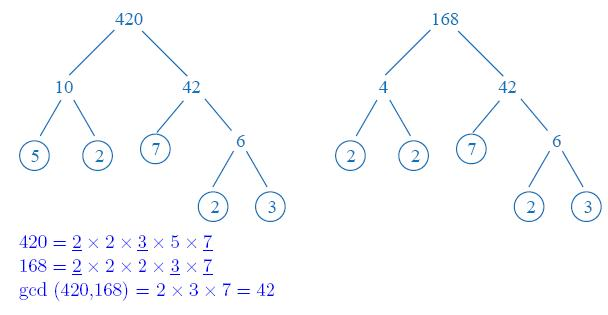# GCD Of Two Numbers In Java – Programs | 5 Ways

Java program to find out the GCD between two numbers. Here, we will discuss the various methods to find out the GCD between two numbers. Also, we’ll learn how to calculate the GCD of n numbers. Soon Compiler has added to it so that you can execute the set of programs yourself. The methods as aforementioned are:

• Using Static Method
• Using While Loop
• Using Functions
• Using Recursion

GCD or Greatest Common Divisor of two or more given numbers is the largest value that divides the given numbers wholly, without leaving any fraction behind.

As in the example shown below, we take two numbers 420 and 168.

After Prime Factorization, we get that

168 = 2 * 2 * 2 * 3 * 7

420 = 2 * 2 * 3 * 5 * 7

The common factors are 2 * 3 * 7 = 42.

Hence, the GCD of 168 and 420 is 42.Source

## Using Static Method

1) Read the values using scanner class method  sc.nextInt(),and store those values in to the variables n1,n2.

If both the numbers n1,n2>0 then call the static method gcdCal(n1,n2) in main method.

2) The n1,n2 values will be passed to a,b and Checks the while condition b>0,if the condition is true then b assigned to temp, b=remainder of b/a, “a” initialized with temp, repeats until the while condition b>0 is false and returns the “a” value which is GCD of two numbers.

Output:

### Using While Loop

1) Read the values using scanner class object and assign those values to the variables x,y.

2) If both the numbers greater than 0, then checks the while condition while(x!=y), if it is true – then if x>y then x=x-y else y=y-x.

3) Repeat until x!=y and returns the x value which is the GCD of two numbers.

Output:

#### Using Functions

1) In this program we have a function long greater(long a, long b), it calculates the GCD of two numbers a,b and returns the number.

Output:

##### Using Recursion

1) In this program greater(long a, long b) calls itself as greater(a,b), the greater method is a recursive method. It repeats until if the condition is false and returns “a” value which is GCD of a,b.

Output:

## Finding GCD for n numbers

1) To store elements into the int array

2) Find GCD of n numbers by passing “r” value and each element of an array to the constructor as GcdCalculation(long a, long b).

Output:

More Java Programs:

x

## Plus Star Pattern Java Program | Patterns

Java program to print plus star pattern program – We have written the below print/draw ...